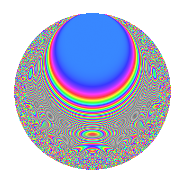Properties

 Label 4009.2.a.eLevel 4009 Weight 2 Character orbit 4009.a Self dual Yes Analytic conductor 32.012 Analytic rank 0 Dimension 82 CM No

Related objects

Newspace parameters

 Level: $$N$$ = $$4009 = 19 \cdot 211$$ Weight: $$k$$ = $$2$$ Character orbit: $$[\chi]$$ = 4009.a (trivial)

Newform invariants

 Self dual: Yes Analytic conductor: $$32.0120261703$$ Analytic rank: $$0$$ Dimension: $$82$$ Fricke sign: $$-1$$ Sato-Tate group: $\mathrm{SU}(2)$

$q$-expansion

The dimension is sufficiently large that we do not compute an algebraic $$q$$-expansion, but we have computed the trace expansion.

 $$\operatorname{Tr}(f)(q) =$$ $$82q$$ $$\mathstrut +\mathstrut 15q^{2}$$ $$\mathstrut +\mathstrut 12q^{3}$$ $$\mathstrut +\mathstrut 89q^{4}$$ $$\mathstrut +\mathstrut 9q^{5}$$ $$\mathstrut +\mathstrut 9q^{6}$$ $$\mathstrut +\mathstrut 14q^{7}$$ $$\mathstrut +\mathstrut 42q^{8}$$ $$\mathstrut +\mathstrut 92q^{9}$$ $$\mathstrut +\mathstrut O(q^{10})$$ $$\operatorname{Tr}(f)(q) =$$ $$82q$$ $$\mathstrut +\mathstrut 15q^{2}$$ $$\mathstrut +\mathstrut 12q^{3}$$ $$\mathstrut +\mathstrut 89q^{4}$$ $$\mathstrut +\mathstrut 9q^{5}$$ $$\mathstrut +\mathstrut 9q^{6}$$ $$\mathstrut +\mathstrut 14q^{7}$$ $$\mathstrut +\mathstrut 42q^{8}$$ $$\mathstrut +\mathstrut 92q^{9}$$ $$\mathstrut +\mathstrut 4q^{10}$$ $$\mathstrut +\mathstrut 41q^{11}$$ $$\mathstrut +\mathstrut 26q^{12}$$ $$\mathstrut +\mathstrut 13q^{13}$$ $$\mathstrut +\mathstrut 22q^{14}$$ $$\mathstrut +\mathstrut 41q^{15}$$ $$\mathstrut +\mathstrut 87q^{16}$$ $$\mathstrut +\mathstrut 12q^{17}$$ $$\mathstrut +\mathstrut 24q^{18}$$ $$\mathstrut +\mathstrut 82q^{19}$$ $$\mathstrut +\mathstrut 26q^{20}$$ $$\mathstrut +\mathstrut 29q^{21}$$ $$\mathstrut +\mathstrut 2q^{22}$$ $$\mathstrut +\mathstrut 59q^{23}$$ $$\mathstrut +\mathstrut 16q^{24}$$ $$\mathstrut +\mathstrut 67q^{25}$$ $$\mathstrut +\mathstrut 24q^{26}$$ $$\mathstrut +\mathstrut 42q^{27}$$ $$\mathstrut -\mathstrut 2q^{28}$$ $$\mathstrut +\mathstrut 101q^{29}$$ $$\mathstrut -\mathstrut 22q^{30}$$ $$\mathstrut +\mathstrut 48q^{31}$$ $$\mathstrut +\mathstrut 69q^{32}$$ $$\mathstrut +\mathstrut 3q^{33}$$ $$\mathstrut +\mathstrut q^{34}$$ $$\mathstrut +\mathstrut 38q^{35}$$ $$\mathstrut +\mathstrut 82q^{36}$$ $$\mathstrut +\mathstrut 16q^{37}$$ $$\mathstrut +\mathstrut 15q^{38}$$ $$\mathstrut +\mathstrut 82q^{39}$$ $$\mathstrut +\mathstrut 20q^{40}$$ $$\mathstrut +\mathstrut 86q^{41}$$ $$\mathstrut -\mathstrut q^{42}$$ $$\mathstrut +\mathstrut 9q^{43}$$ $$\mathstrut +\mathstrut 82q^{44}$$ $$\mathstrut -\mathstrut 8q^{45}$$ $$\mathstrut +\mathstrut 43q^{46}$$ $$\mathstrut +\mathstrut 24q^{47}$$ $$\mathstrut +\mathstrut 34q^{48}$$ $$\mathstrut +\mathstrut 76q^{49}$$ $$\mathstrut +\mathstrut 82q^{50}$$ $$\mathstrut +\mathstrut 57q^{51}$$ $$\mathstrut -\mathstrut 22q^{52}$$ $$\mathstrut +\mathstrut 39q^{53}$$ $$\mathstrut +\mathstrut 17q^{54}$$ $$\mathstrut -\mathstrut 21q^{55}$$ $$\mathstrut +\mathstrut 50q^{56}$$ $$\mathstrut +\mathstrut 12q^{57}$$ $$\mathstrut +\mathstrut 33q^{58}$$ $$\mathstrut +\mathstrut 79q^{59}$$ $$\mathstrut +\mathstrut 87q^{60}$$ $$\mathstrut +\mathstrut 4q^{61}$$ $$\mathstrut +\mathstrut 40q^{62}$$ $$\mathstrut +\mathstrut 44q^{63}$$ $$\mathstrut +\mathstrut 90q^{64}$$ $$\mathstrut +\mathstrut 66q^{65}$$ $$\mathstrut -\mathstrut 39q^{66}$$ $$\mathstrut +\mathstrut 33q^{67}$$ $$\mathstrut -\mathstrut 9q^{68}$$ $$\mathstrut +\mathstrut 60q^{69}$$ $$\mathstrut +\mathstrut 30q^{70}$$ $$\mathstrut +\mathstrut 168q^{71}$$ $$\mathstrut +\mathstrut 15q^{72}$$ $$\mathstrut -\mathstrut 28q^{73}$$ $$\mathstrut +\mathstrut 35q^{74}$$ $$\mathstrut +\mathstrut 55q^{75}$$ $$\mathstrut +\mathstrut 89q^{76}$$ $$\mathstrut +\mathstrut 19q^{77}$$ $$\mathstrut -\mathstrut 41q^{78}$$ $$\mathstrut +\mathstrut 121q^{79}$$ $$\mathstrut +\mathstrut 64q^{80}$$ $$\mathstrut +\mathstrut 110q^{81}$$ $$\mathstrut +\mathstrut 41q^{82}$$ $$\mathstrut +\mathstrut 28q^{84}$$ $$\mathstrut +\mathstrut 17q^{85}$$ $$\mathstrut +\mathstrut 80q^{86}$$ $$\mathstrut +\mathstrut 29q^{87}$$ $$\mathstrut +\mathstrut 49q^{88}$$ $$\mathstrut +\mathstrut 83q^{89}$$ $$\mathstrut -\mathstrut 42q^{90}$$ $$\mathstrut +\mathstrut 38q^{91}$$ $$\mathstrut +\mathstrut 71q^{92}$$ $$\mathstrut -\mathstrut q^{93}$$ $$\mathstrut +\mathstrut 89q^{94}$$ $$\mathstrut +\mathstrut 9q^{95}$$ $$\mathstrut +\mathstrut 35q^{96}$$ $$\mathstrut -\mathstrut 23q^{97}$$ $$\mathstrut +\mathstrut 135q^{98}$$ $$\mathstrut +\mathstrut 93q^{99}$$ $$\mathstrut +\mathstrut O(q^{100})$$

Embeddings

For each embedding $$\iota_m$$ of the coefficient field, the values $$\iota_m(a_n)$$ are shown below.

For more information on an embedded modular form you can click on its label.

Label $$a_{2}$$ $$a_{3}$$ $$a_{4}$$ $$a_{5}$$ $$a_{6}$$ $$a_{7}$$ $$a_{8}$$ $$a_{9}$$ $$a_{10}$$
1.1 −2.71228 −0.799829 5.35647 2.45685 2.16936 −1.47623 −9.10370 −2.36027 −6.66368
1.2 −2.64355 −0.244924 4.98837 −2.38993 0.647469 −3.32754 −7.89992 −2.94001 6.31792
1.3 −2.62936 3.14041 4.91352 2.46626 −8.25726 1.15889 −7.66068 6.86218 −6.48468
1.4 −2.62201 −2.46509 4.87496 −1.74208 6.46350 −2.61324 −7.53817 3.07667 4.56776
1.5 −2.40525 0.482964 3.78524 1.80217 −1.16165 −1.90696 −4.29395 −2.76675 −4.33466
1.6 −2.39399 1.52449 3.73121 −2.51919 −3.64962 −1.00997 −4.14450 −0.675931 6.03093
1.7 −2.39206 −0.112128 3.72195 0.840987 0.268217 2.66760 −4.11902 −2.98743 −2.01169
1.8 −2.27080 −1.94834 3.15654 0.0772315 4.42430 2.91283 −2.62627 0.796044 −0.175377
1.9 −2.22938 2.96636 2.97012 −0.893171 −6.61314 4.38195 −2.16277 5.79930 1.99121
1.10 −2.21501 1.93892 2.90626 3.78369 −4.29473 0.133386 −2.00737 0.759427 −8.38090
1.11 −2.16486 3.17577 2.68660 0.570979 −6.87507 −2.83752 −1.48639 7.08548 −1.23609
1.12 −2.09995 −2.73690 2.40979 2.29135 5.74735 −1.74327 −0.860544 4.49062 −4.81171
1.13 −1.93317 −1.52394 1.73714 −2.99074 2.94604 −0.667472 0.508147 −0.677595 5.78161
1.14 −1.90886 1.35744 1.64375 −1.48428 −2.59115 2.77982 0.680038 −1.15737 2.83328
1.15 −1.89977 −2.93667 1.60912 −0.296578 5.57898 2.59029 0.742589 5.62400 0.563430
1.16 −1.69115 −1.97765 0.859975 −4.28235 3.34450 4.00531 1.92795 0.911117 7.24208
1.17 −1.66851 −1.79206 0.783934 2.47432 2.99007 0.566931 2.02902 0.211475 −4.12843
1.18 −1.53574 2.00602 0.358512 1.47205 −3.08073 −4.88163 2.52091 1.02410 −2.26069
1.19 −1.53259 1.29314 0.348820 3.28950 −1.98185 4.48074 2.53058 −1.32779 −5.04144
1.20 −1.51298 0.189655 0.289107 −0.503823 −0.286944 −1.74123 2.58855 −2.96403 0.762274
See all 82 embeddings
 $$n$$: e.g. 2-40 or 990-1000 Embeddings: e.g. 1-3 or 1.82 Significant digits: Format: Complex embeddings Normalized embeddings Satake parameters Satake angles

Inner twists

This newform does not have CM; other inner twists have not been computed.

Atkin-Lehner signs

$$p$$ Sign
$$19$$ $$-1$$
$$211$$ $$1$$

Hecke kernels

This newform can be constructed as the kernel of the linear operator $$T_{2}^{82} - \cdots$$ acting on $$S_{2}^{\mathrm{new}}(\Gamma_0(4009))$$.